Courses

# Test: Simpler Notations

## 10 Questions MCQ Test Theory of Computation | Test: Simpler Notations

Description
This mock test of Test: Simpler Notations for Computer Science Engineering (CSE) helps you for every Computer Science Engineering (CSE) entrance exam. This contains 10 Multiple Choice Questions for Computer Science Engineering (CSE) Test: Simpler Notations (mcq) to study with solutions a complete question bank. The solved questions answers in this Test: Simpler Notations quiz give you a good mix of easy questions and tough questions. Computer Science Engineering (CSE) students definitely take this Test: Simpler Notations exercise for a better result in the exam. You can find other Test: Simpler Notations extra questions, long questions & short questions for Computer Science Engineering (CSE) on EduRev as well by searching above.
QUESTION: 1

### Given Language: L= {xϵ∑= {a, b} |x has a substring ‘aa’ in the production}. Which of the corresponding representation notate the same?

Solution:

The states transited has been written corresponding to the transitions as per the row and column. The row represents the transitions made and the ultimate.

QUESTION: 2

### the row and column. The row represents the transitions made and the ultimate.2.Let u=’1101’, v=’0001’, then uv=11010001 and vu= 00011101.Using the given information what is the identity element for the string?

Solution:

Identity relation: εw = wε = w, thus the one satisfying the given relation will be the identity element.

QUESTION: 3

### Which of the following substring will the following notation result?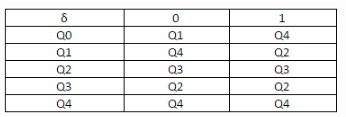Solution:

The given DFA notation accepts the string of even length and prefix ‘01’.

QUESTION: 4

Predict the following step in the given bunch of steps which accepts a strings which is of even length and has a prefix=’01’
δ (q0, ε) =q0 < δ(q0,0) =δ (δ (q0, ε),0) =δ(q0,0) =q1 < _______________

Solution:

Here, δ refers to transition function and results into new state or function when an transition is performed over its state.

QUESTION: 5

Fill the missing blank in the given Transition Table:
Language L= {xϵ∑= {0,1} |x accepts all the binary strings not divisible by 3}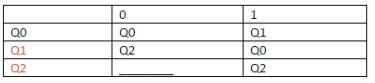Solution:

The tabular representation of DFA is quite readable and can be used to some ore complex problems. Here, we need to form the transition graph and fill up the given blank..

QUESTION: 6

.Which among the following is the missing transition in the given DFA?
L= {xϵ∑= {a, b} | x starts with a and ends with b}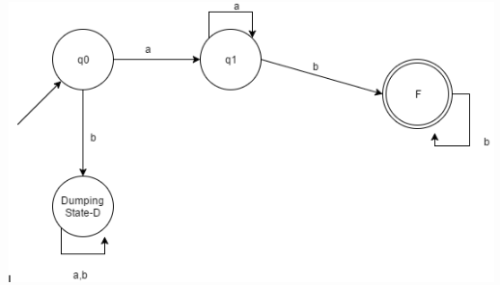Solution:

For the given Language, the transition missing is δ (F, a) =q1.

QUESTION: 7

The complement of a language will only be defined when and only when the __________ over the language is defined.

Solution:

It is not possible to define the complement of a language without defining the input alphabets. Example: A language which does not consist of substring ‘ab’ while the complement would be the language which does contain a substring ‘ab’.

QUESTION: 8

Which among the following is not notated as infinite language?

Solution:

Factorial, here is the most appropriate non-infinite domain. Otherwise, palindrome and reverse have infinite domains.

QUESTION: 9

Which among the following states would be notated as the final state/acceptance state?L= {xϵ∑= {a, b} | length of x is 2}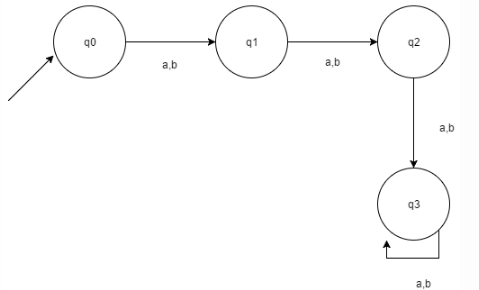Solution:

According to the given language, q2 Is to become the final/acceptance state in order to satisfy.

QUESTION: 10

Which of the following are the final states in the given DFA according to the Language given.?
L= {xϵ∑= {a, b} |length of x is at most 2}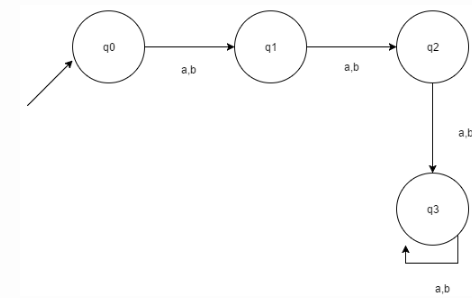Solution:

According to the given language, the length is at most 2, thus the answer is found accordingly.